## Computational Fluid Dynamics and Vortex Dynamics

The interaction between flapping wings and unsteady flows is governed by the Navier-Stokes equation coupled to the dynamic boundaries. The typical Reynolds number for insect flight is in the range of 10-10000, where both the viscous and inertial forces are relevant. While some of the most interesting fluid dynamics originates near a moving sharp interface, computational schemes typically encounter great difficulty in resolving moving interfaces, a known difficulty in nearly all fluid-structure simulations.

We have improved a number of computational methods and developed new algorithms for solving the Navier-Stokes equation coupled to moving interfaces. The first set of codes are Navier-Stokes solvers for simulating a 2D rigid flapping wing, based on an essentially compact 4th order finite difference scheme in vorticity and stream function formulation. In these solvers we took advantage of coordinate transformations and conformal mapping to resolve sharp wing tips so as to avoid grid-regeneration. With these methods, we have investigated the unsteady aerodynamics of forward and hovering flight as well as of plates freely falling in a fluid. To simulate multiple bodies, we have further developed a Cartesian method coupled to an overset grid that are attached to the moving geometries.

To go beyond 2D simulations of rigid objects, we have recently developed a more general purpose code for simulating multiple flexible freely objects. The main advance in the new immersed interface method is to obtain a 2nd order accuracy of the sharp moving surface at an intermediate range of Reynolds flows. To avoid introducing ad-hoc boundary conditions at the moving interface, we derived systematically from the 3D Navier-Stokes equation the jump conditions on the fluid variables caused by the singular force. Our analysis showed that a 2nd order accuracy along the sharp interface requires jump conditions on derivatives of velocity that are of higher order than those appearing in the principal jump conditions. In addition, the temporal jump conditions must be included in order to have a correct scheme. To handle the spatial and temporal jump conditions in the finite difference scheme, we derived a generalized Taylor expansions for functions with discontinuity of an arbitrary order.

This code has been adapted to studies of passive wing pitching in insect flight. Most recently, the code has been further developed to simulate hydrodynamic interactions of multiple objects at intermediate Reynolds numbers.

### A New method for computing particle collisions in Navier-Stokes flows Journal of Computational Physics (2019) [PDF]

Particle collisions in fluids are ubiquitous, but to compute the collision dynamics in a Navier-Stokes flow remains challenging. In addition to capturing the two-way coupling between the fluid and the particles, a key difficulty is to resolve the collision dynamics mediated by the flow. The gap between particles during collision is minuscule. This introduces a small length scale which needs to be resolved simultaneously with the flow at the large scale. Our goal is to develop a numerical scheme that is accurate and efficient in computing the Navier-Stokes flow around moving particles while taking into account the effect of the lubrication forces on the collisions. Our method integrates the immersed interface method with the lubrication theory in a way that directly couples all three parts, the bulk flow, the flow in the gap, and the dynamics of the freely moving particles. We present a general algorithm for computing the collision. To test the method, we study four fundamental cases involving normal and tangential collisions, so that we can compare numerics against analytic solutions in the lubrication layer. In addition, we provide the lubrication solution needed for computing collisions between surfaces of any shapes in arbitrary relative motions, so that the method can be applied to other cases.

### The effect of gravity and dimensionality on the impact of cylinders and spheres onto a wall in a viscous fluid Physics of Fluid (2017) [PDF]As a solid body approaches a wall in a viscous fluid, the flow in the gap between them is dominated by the viscous effect and can be approximated by the lubrication theory. Here we show that without gravity, a cylinder comes to rest asymptotically at a finite separation from the wall, whereas with gravity, the cylinder approaches the wall asymptotically and contact does not happen in finite time. A cylinder approaches the wall much slower compared to a sphere under matching conditions, implying that the lubrication approximates hold longer before the molecular scale sets in. Our results further serve as a building block for analyzing particle interactions in close proximity, and provide analytic results for integrating the lubrication theory into the computations of Navier-Stokes equations.

### A. El Yacoubi, S. Xu, and Z. J. Wang, Computational Study of the Interaction of Freely Moving Particles at Intermediate Reynolds Numbers, Journal of Fluid Mechanics (2012) [PDF]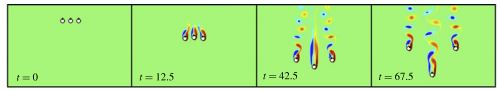Motivated by our interest in understanding collective behaviour and self-organization resulting from hydrodynamic interactions, we investigate the two-dimensional dynamics of horizontal arrays of settling cylinders at intermediate Reynolds numbers. To simulate these dynamics, we develop a direct numerical simulation based on the immersed interface method. A novel aspect of our method is its ability to efficiently and accurately couple the dynamics of the freely moving objects with the fluid. We report the falling configuration and the wake pattern of the array, and investigate their dependence on the number of particles, n, as well as the initial inter-particle spacing, d0. We find that, in the case of odd-numbered arrays, the middle cylinder is always leading, whereas in the case of even-numbered arrays, the steady-state shape is concave-down. In large arrays n>=5, the outer pairs tend to cluster. In addition, we analyse detailed kinematics, wakes and forces of three settling cylinders. We find that the middle one experiences a higher drag force in the presence of neighbouring cylinders, compared to an isolated settling cylinder, resulting in a decrease in its settling velocity. For a small initial spacing d0, the middle cylinder experiences a strong sideway repulsive force, the magnitude of which increases with decreasing d0. During the fall, the left and right cylinders rotate outwards and shed vortices in anti-phase.

### Immersed Interface Method 3D Implementation, Computer Methods in Applied Mechanics and Engineering (2007) [PDF]

In immersed interface methods, solids in a fluid are represented by singular forces in the Navier-Stokes equations, and flow jump conditions induced by the singular forces directly enter into numerical schemes. This paper focuses on the implementation of an immersed interface method for simulating fluid-solid interaction in 3D. The method employs the MAC scheme for the spatial discretization, the RK4 scheme for the time integration, and an FFT-based Poisson solver for the pressure Poisson equation. A fluid-solid interface is tracked by Lagrangian markers. Intersections of the interface with MAC grid lines identify finite difference stencils on which jump contributions to finite difference schemes are needed. To find the intersections and to interpolate jump conditions from the Lagrangian markers to the intersections, parametric triangulation of the interface is used. The velocity of the Lagrangian markers is interpolated directly from surrounding MAC grid nodes with interpolation schemes accounting for jump conditions. Numerical examples demonstrate that (1) the method has near second-order accuracy in the infinity norm for velocity, and the accuracy for pressure is between first and second order; (2) the method conserves the volume enclosed by a no-penetration boundary; and (3) the method can efficiently handle multiple moving solids with ease.

### Immersed Interface Method 2D Implementation, Journal of Computational Physics (2006) [PDF]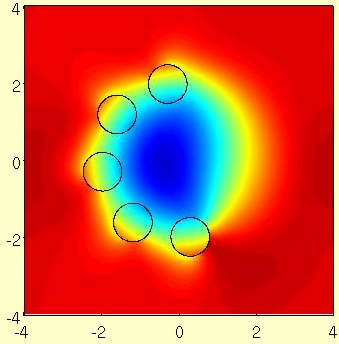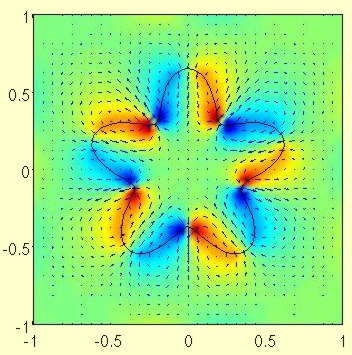In the immersed interface method, boundaries are represented as singular force in the Navier-Stokes equations, which enters a numerical scheme as jump conditions. Recently, we systematically derived all the necessary spatial and temporal jump conditions for simulating incompressible viscous flows subject to moving boundaries in 3D with second-order spatial and temporal accuracy near the boundaries [Sheng Xu, Z. Jane Wang, Systematic derivation of jump conditions for the immersed interface method in three-dimensional flow simulation, SIAM J. Sci. Comput., 2006, in press]. In this paper we implement the immersed interface method to incorporate these jump conditions in a 2D numerical scheme. We study the accuracy, efficiency and robustness of our method by simulating Taylor-Couette flow, flow induced by a relaxing balloon, flow past single and multiple cylinders, and flow around a flapping wing. Our results show that: (1) our code has second-order accuracy in the infinity norm for both the velocity and the pressure; (2) the addition of an object introduces relatively insignificant computational cost; (3) the method is equally effective in computing flow subject to boundaries with prescribed force or boundaries with prescribed motion.

### Immersed Interface Method Systematic Derivation of Jump Conditions, SIAM Journal on Scientific Computing (2006) [PDF]

In this paper, we systematically derive jump conditions for the immersed interface method [SIAM J. Numer. Anal., 31 (1994), pp. 1019-1044; SIAM J. Sci. Comput., 18 (1997), pp.709-735] to simulate three-dimensional incompressible viscous flows subject to moving surfaces. The surfaces are represented as singular forces in the Navier-Stokes equations, which give rise to discontinuities of flow quantities. The principal jump conditions across a closed surface of the velocity, the pressure, and their normal derivatives have been derived by Lai and Li [Appl. Math. Lett., 14 (2001), pp. 149-154]. In this paper, we first extend their derivation to generalized surface parametrization. Starting from the principal jump conditions, we then derive the jump conditions of all first-, second-, and third-order spatial derivatives of the velocity and the pressure. We also derive the jump conditions of first- and second-order temporal derivatives of the velocity. Using these jump conditions, the immersed interface method is applicable to the simulation of three-dimensional incompressible viscous flows subject to moving surfaces, where near the surfaces the first- and second order spatial derivatives of the velocity and the pressure can be discretized with, respectively, third- and second-order accuracy, and the first-order temporal derivatives of the velocity can be discretized with second-order accuracy.

### Multiple-Wing Interaction: Vorticity and Stream Function Formulation Journal of Computational Physics (2003) [PDF]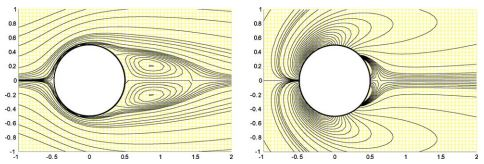To explore the possible benefit of multiple wing interactions, it is desirable to have an efficient computational tool to simulate multiple bodies moving in fluids. The existing methods involving grid regeneration or overset grids are intrinsically complicated. We developed a new algorithm for handing general fluid and solid body interactions, which is a two dimensional Cartesian grid method that treats the multiple objects as embedded discontinuities. The method uses O(N*ln(N)+M) per operation step, where N is the number of nodes in the regular Cartesian grid and M is the number of nodes in the immersed object surface discretization. The method is tested in the canonical examples of flow past cylinder and interactions of two cylinders, and now is being used to simulate flapping motions of dragonflies obtained in our recent experiments of tethered flight.

### Far Field Boundary Conditions Journal of Computational Physics (1999) [PDF]

Solving flows numerically in open geometry requires specification of flows at some finite computational boundary. I find a simple scheme for implementing the far-field boundary condition exactly for the Poisson equation with source term of finite support. This numerical scheme avoids introducing mixed boundary conditions in the far field, thus it can be used efficiently with FFT.

### Connection between corner vortices and shear layer instability in flow past an ellipse Physics of Fluids (1999) [PDF]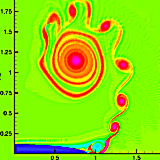We investigate shear instabilities leading to secondary vortices in flow past an ellipse at high Reynolds numbers (Re=104) by direct numerical simulation. We find that the temporal and spatial periodicities in the shear layer are independent of the numerical resolutions. More interestingly, the turnover time of the corner vortex coincides with the periodicity of the vortex roll-up in the shear layer. We hypothesize that the corner vortex acts as a rotor for triggering the instabilities.